# Query Evaluation Flow¶

## Definitions¶

### Clause¶

We analyze Expression Trees and label tree nodes with Clauses.

A Clause is a boolean condition that can be applied to a data subset (i.e, object) $$S$$, typically by inspecting its metadata. For a Clause $$c$$ and a (boolean) query expression $$e$$, we say that $$c$$ represents $$e$$ (denoted by $$c \wr e$$ ), if for every object $$S$$, whenever there exists a row $$r \in S$$ that satisfies $$e$$, then $$S$$ satisfies $$c$$. This means that if $$S$$ does not satisfy $$c$$, then $$S$$ can be safely skipped when evaluating the query expression $$e$$.

For example, given the expression $$e = temp > 101$$ the clause $$c = \max_{r \in S} temp(r) > 101$$ represents $$e$$ (denoted by $$c \wr e$$ ). Therefore, objects where $$c = \max_{r \in S} temp(r) <= 101$$ can be safely skipped

### Filter¶

The labeling process of Expression Trees is done using filters. An algorithm A is a filter if it performs the following action: When given an expression tree $$e$$ as input, for every (boolean valued) vertex $$v$$ in $$e$$, it adds a set of clauses $$C$$ s.t $$\forall c \in C$$: $$c \wr v$$.

For example, given the expression $$e = temp > 101$$: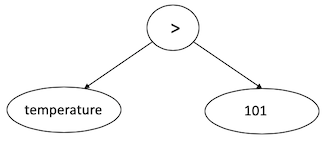A filter $$f$$ might label the Expression Tree using a MaxClause: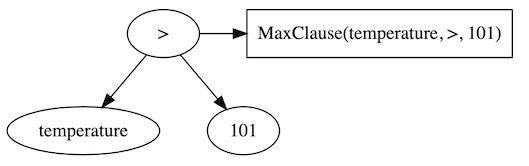MaxClause(c,>,v) is defined as $$c = \max_{r \in S} c(r) > v$$ Where for a c is the column name v is the value. Since MaxClause(temperature,>,101) represents the node to which it was applied, $$f$$ acted as a filter.

## Clause Translator¶

A component which translates a Clause to a specific implementation according to the metadatastore type.

## Query Evaluation Flow¶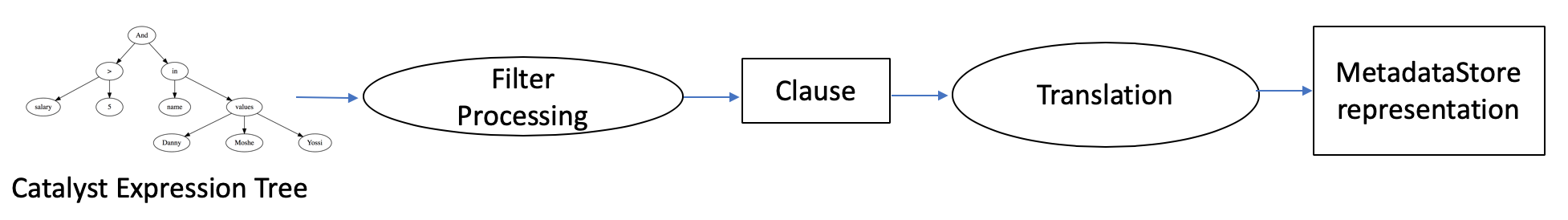Query evaluation is done in 2 phases:

1. A query’s Expression Tree $$e$$ is labelled using a set of clauses

1. The clauses are combined to provide a single clause which represents $$e$$.

2. The labelling process is extensible, allowing for new index types and UDFs.

2. The clause is translated to a form that can be applied at the metadata store to filter out objects which can be skipped during query run time.

### A simple example¶

For example, given the query:

SELECT *
FROM employees
WHERE salary > 5 AND
name IN (‘Danny’, ’Moshe’, ’Yossi’)


The Expression Tree can be visualized as following: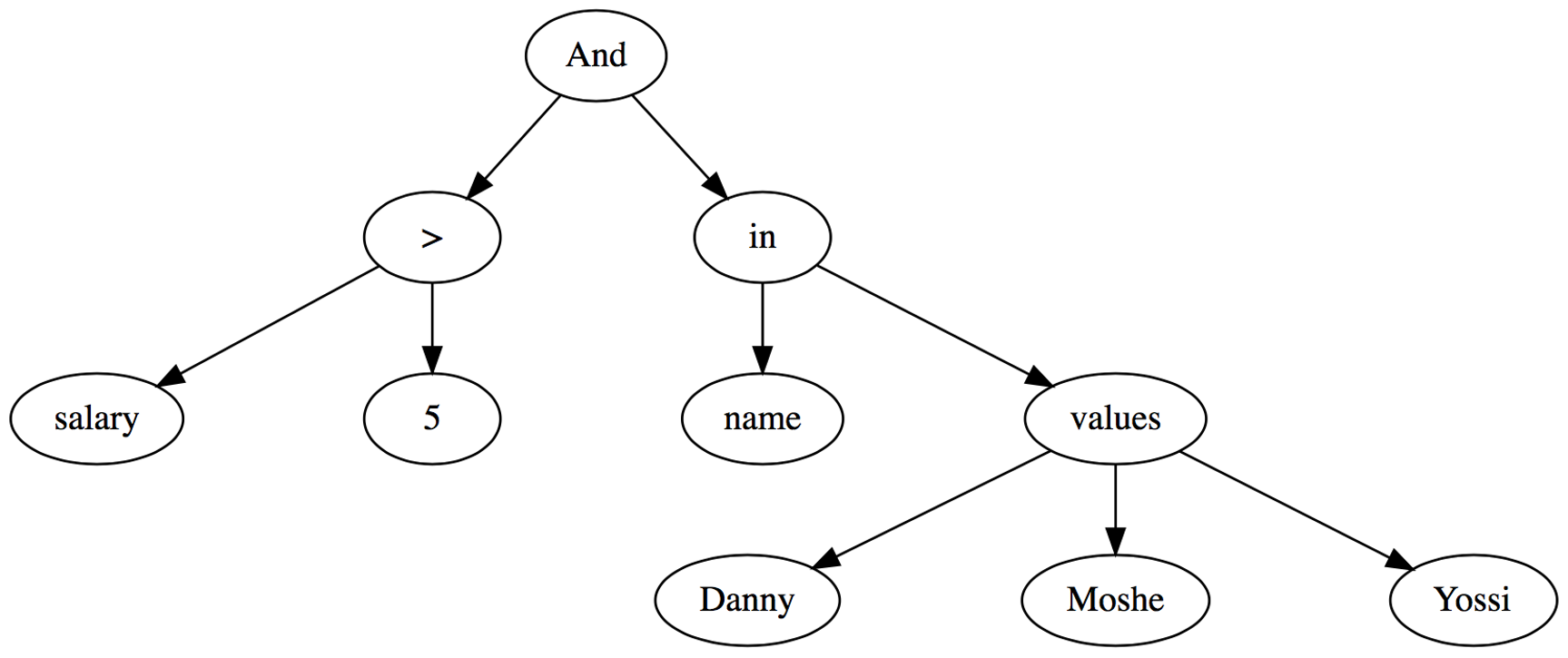Assuming we have a MinMax Index for the salary column (store minimum and maximum values for each object) and a ValueList Index on the name column (storing the distinct list of values for each object).

• Applying the MinMax filter results in:• Applying the ValueList filter on the results of the previous filter results in: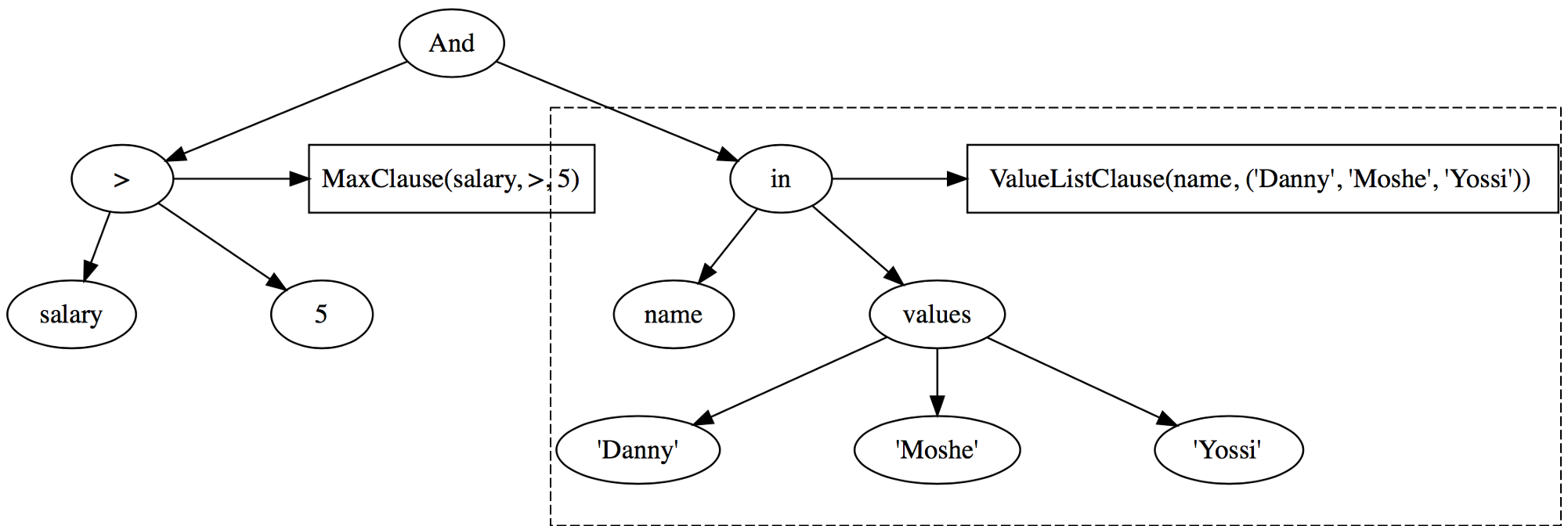• Finally we generate a combined Abstract Clause:
AND(MaxClause(salary, >, 5),ValueListClause(name, ('Danny', 'Moshe', 'Yossi')))


This clause will be translated to a form that can be applied at the metadata store to filter out objects which can be skipped during query run time